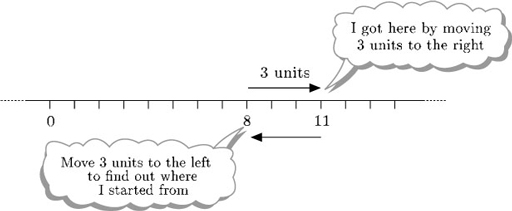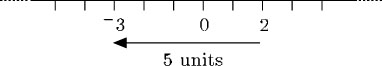Science, Maths & Technology

### Become an OU studentNumbers, units and arithmetic

Start this free course now. Just create an account and sign in. Enrol and complete the course for a free statement of participation or digital badge if available.

# 3.15.1 Subtraction on the number line

Now what about subtraction? You can think of subtraction as undoing addition: adding 3 to 8 gets you 11, and so subtracting 3 from the answer, 11, gets you back to 8. Therefore, in terms of the number line, subtracting 3 from 11 means starting at 11 and moving 3 units to the left.So 11 − 3 = 8. Subtracting a positive number means moving to the left along the number line. Thinking in terms of the contents of the piggy bank: if Thomas had £11 in his piggy bank and subtracts £3 (to buy a toy), he is left with £8.

Suppose on one occasion Thomas has £2 in his piggy bank. He wants to buy a toy for £5. 2 − 5 means starting at 2 and moving 5 units to the left.This takes you back to 3, so 2 − 5 = 3.

So Thomas needs to borrow £3 and gets an IOU (from his mother) which he puts in his piggy bank. His piggy bank is worth £3.